Disclaimer: This is an example of a student written essay.

Any scientific information contained within this essay should not be treated as fact, this content is to be used for educational purposes only and may contain factual inaccuracies or be out of date.

# Reality of Unification Theory

 ✅ Paper Type: Free Essay ✅ Subject: Physics ✅ Wordcount: 3742 words ✅ Published: 30th Jan 2018

1. Introduction

Unification means: finding the similarities, finding the limiting physical constants, finding the key numbers, coupling the key physical constants, coupling the key physical concepts, coupling the key physical properties, minimizing the number of dimensions, minimizing the number of inputs. This is a very lengthy process. In all these cases observations, interpretations, experiments and imagination play a key role. The main difficulty is with interpretations and observations. At fundamental level understanding the observed new coincidences and confirming the observed coincidences seem to be a very tough job. Constructing semi empirical relations among the physical constants of various interdisciplinary branches of physics with all possible interpretations may help in resolving the issues. Which way/method is the best – will be decided by future experiments, observations and interpretations. As it is interconnected with all branches of physics, ‘semi empirical approach’ seems be the easiest and shortcut way. It sharpens and guides human thinking ability in understanding the reality of unification. For any theoretical concept or mathematical model or semi empirical relation, ‘workability’ is more important than its inner beauty and ‘workability’ is the base of any semi empirical approach.

In this paper by introducing the unified mass unit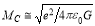the authors made an attempt to fit and understand the origin of the nuclear charge radius, the Planck’s constant and the strong coupling constant in a unified approach that connects gravity and strong interaction via the electromagnetic and gravitational force ratio of proton and electron. Finally by considering the proton rest energy and the nuclear charge radius the authors made an attempt to fit the semi empirical mass formula energy coefficients in a very simple way.

1. To fit the nuclear charge radius, the Planck’s constant and the strong coupling constant

It is well known that e, c and G play a vital role in fundamental physics. With these 3 constants space-time curvature concepts at a charged particle surface can be studied. Similar to the Planck mass an interesting unified mass unit can be constructed in the following way.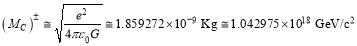(1)

Note that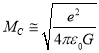plays a crucial role in microscopic physics as well cosmology. It is having a long history. It was first introduced by the physicist George Johnstone Stoney . He is most famous for introducing the term ‘electron’as the ‘fundamental unit quantity of electricity’. With this mass unit in unification program with a suitable proportionality it may be possible to represent the characteristic mass of any elementary charge. It can be considered as the seed of galactic matter or galactic central black hole. It can also be considered as the seed of any cosmic structure. If 2 such oppositely charged particles annihilates, a large amount of energy can be released. It is well assumed that free space is a reservoir for pair particles creation. If so under certain extreme conditions at the vicinity of massive stars or black holes, a very high energy radiation can be seen to be emitted by the virtue of pair annihilation of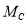. Note that the basic concept of unification is to understand the origin of ‘mass’ of any particle. Mass is the basic property in ‘gravitation’ and charge is the basic property in ‘atomicity’. So far no model established a cohesive relation in between ‘electric charge’ and ‘mass’ of any ‘elementary particle’. From astrophysics point of view the fundamental questions to be answered are: 1) Without charge, is there any independent existence to ‘mass’ of any star? 2) Is black hole – a neutral body or electrically a neutralized body? To understand these questions the authors made an attempt to construct the above unified mass unit.

The subject of final unification is having a long history. After the nucleus was discovered in 1908, it was clear that a new force was needed to overcome the electrostatic repulsion of the positively charged protons. Otherwise the nucleus could not exist. Moreover, the force had to be strong enough to squeeze the protons into a volume of size 10−15 meter. In general the word‘strong’is used since the strong interaction is the “strongest” of the four fundamental forces. Its observed strength is around 102 times that of the electromagnetic force, some 105times as great as that of the weak force, and about 10 39 times that of gravitation. The aim of unification is to understand the relation that connects ‘gravity’, ‘mass’, ‘charge’ and the ‘microscopic space-time curvature’. Many scientists addressed this problem in different ways [2-4]. The authors also made many attempts in their previously published papers [5-11]. Experimentally observed nuclear charge radius [12-15] can be fitted with the following strange and simple unified relation.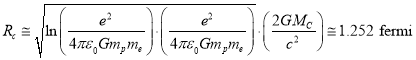(2)

Here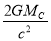can be considered as the Schwarzschild radius [16,17] of the proposed new mass unit.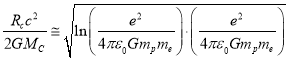(3)

Whether the expression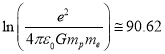playing a ‘key unified role’ or ‘only a fitting role’ to be confirmed. With a great accuracy the famous Planck’s constant can be fitted with the following relation.(4)

Recommended value of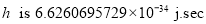and the error is 0.189%. From relations (1) and (2) above relation can be simplified into the following simple form.(5)

Comparing this with the standard definition,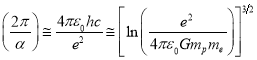(6)

where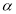is the fine structure ratio.

Let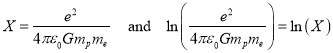(7)

Proceeding further qualitatively and quantitatively currently believed strong coupling constant  can be fitted with the following relation.(8)

Its recommended value is 0.11847 and uncertainty is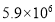ppb. Now the characteristic nuclear force can be expressed as follows.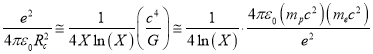(9)

Note that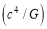can be considered as the limiting magnitude of any kind of force. Similarly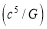can be considered as the limiting magnitude of any kind of power [1,20,21].

1. To fit and co-relate the semi empirical mass formula energy coefficients

In nuclear physics, the semi-empirical mass formula is used to approximate the mass and various other properties of an atomic nucleus. As the name suggests, it is based partly on theory and partly on empirical measurements [23-24]. The theory is based on the liquid drop model proposed by George Gamow, which can account for most of the terms in the formula and gives rough estimates for the values of the coefficients. It was first formulated in 1935 by German physicist Carl Friedrich von Weizsacker, and although refinements have been made to the coefficients over the years, the structure of the formula remains the same today. In the following formulae, let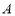be the total number of nucleons, Z the number of protons, and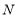the number of neutrons. The mass of an atomic nucleus is given by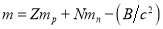(10)

where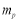and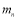are the rest mass of a proton and a neutron, respectively, and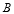is the binding energy of the nucleus. The semi-empirical mass formula states that the binding energy will take the following form.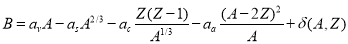(11)

Its modern representation is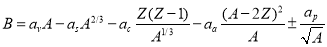(12)

Here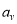= volume energy coefficient,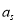is the surface energy coefficient,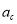is the coulomb energy coefficient,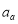is the asymmetry energy coefficient and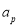is the pairing energy coefficient. By maximizing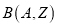with respect to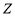, one can find the number of protonsof the stable nucleus of atomic weight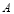as,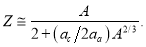(13)

This is roughly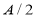for light nuclei, but for heavy nuclei there is an even better agreement with nature. Now with the following two energy units semi empirical mass formula energy coefficients can be fitted in the following way.

Let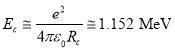(14)

where,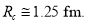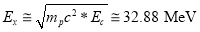(15)

1. The coulombic energy coefficient can be expressed as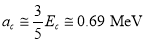(16)

1. The asymmetry energy coefficient can be expressed as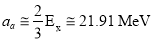(17)

1. The pairing energy coefficient can be expressed as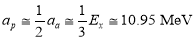(18)

1. The surface energy coefficient can be expressed as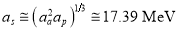(19)

1. The volume energy coefficient can be expressed as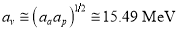(20)

Thus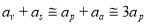(21)

For light and heavy atoms (including super heavy stable isotopes), proton-nucleon stability relation can be expressed with the following semi empirical relation. Clearly speaking by considering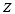its corresponding stable mass number can be estimated directly.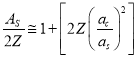(22)

where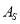can be considered as the stable mass number of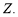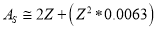(23)

Please see table-1 for fitting the proton number and its corresponding stable mass number.

Table-1: To fit the stable mass numbers of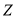S.No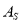1 21 44.8 2 29 63.3 3 37 82.6 4 47 107.9. 5 53 123.9 6 60 142.8 7 69 168.0 8 79 197.3 9 83 209.4 10 92 237.3 11 100 263.0 12 112 303.0 13 118 323.7

See table-2 for the comparison of the semi empirical mass formula energy coefficients.

See table-3 for the calculated semi empirical mass formula nuclear binding energy.

Table-2: Existing and proposed SEMF binding energy coefficients

 Existing energy coefficients Proposed energy coefficients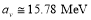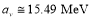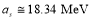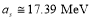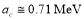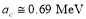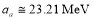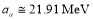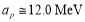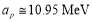Table-3: To fit the SEMF binding energy with the proposed energy coefficients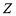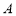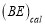in MeV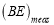in MeV 26 56 490.9 492.254 28 62 544.8 545.259 34 84 725.3 727.341 50 118 1005.6 1004.950 60 142 1184.3 1185.145 79 197 1562.9 1559.40 82 208 1634.8 1636.44 92 238 1818.9 1801.693
1. Discussion and conclusions

The main object of unification is to understand the origin of elementary particles rest mass, magnetic moments and their forces. Right now and till today ‘string theory’ with 4 + 6 extra dimensions not in a position to explain the unification of gravitational and non-gravitational forces. More clearly speaking it is not in a position to bring down the Planck scale to the nuclear size. Note that general relativity does not throw any light on the ‘mass generation’ of charged particles. It only suggests that space-time is curved near the massive celestial objects. More over it couples the cosmic (dust) matter with geometry. But how matter/dust is created? Why and how elementary particle possesses

both charge and mass? Such types of questions are not being discussed in the frame work of general relativity. The first step in unification is to understand the origin of the rest mass of a charged elementary particle. Second step is to understand the combined effects of its electromagnetic (or charged) and gravitational interactions. Third step is to understand its behavior with surroundings when it is created. Fourth step is to understand its behavior with cosmic space-time or other particles. Right from its birth to death, in all these steps the underlying fact is that whether it is a strongly interacting particle or weakly interacting particle, it is having some rest mass. To understand the first two steps somehow one can implement the gravitational constant in sub atomic physics. In this regardcan be considered as the nature’s given unified mass unit. To bring down the Planck mass scale to the observed elementary particles mass scale certainly a large scale factor is required. In this regard, the electromagnetic and gravitational force ratio of proton and electron can be considered as the nature’s given universal scale factor. Thinking positively the proposed relations for fitting the nuclear charge radius, the Planck’s constant and strong the coupling constant can be considered for further analysis positively.

View all

## DMCA / Removal Request

If you are the original writer of this essay and no longer wish to have your work published on UKEssays.com then please:

Related Services

Prices from

BHD57

Approximate costs for:

• 1000 words
• 7 day deliveryHumanity University

Dedicated to your worth and value as a human being!

Related Lectures